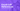# Excel Cell References

May 06, 2012

When writing Excel formulas, you’ll definitely be making references to other cells (like =SUM(A1:A3)). If you haven’t heard of absolute cell references and relative cell references, you’re going to want to read on, because this knowledge can save your life! (nah, it probably won’t :) but it will save you a lot of headaches!)

I touched on cell references previously, however in this post I would like to go further in-depth on the subject. In this post, we’ll be talking about:

• What are cell references?
• Relative cell references
• Absolute cell references
• Keyboard shortcut to toggle between absolute and relative references

## What are cell references?

Let’s get a base of what cell references actually are. A cell reference is an area on your worksheet that you are referring to in your formulas. For example, if you have a simple `SUM()` formula like this:

``=SUM(B2:C5)``

Then `B2:C5` is referencing the cells in the worksheet from `B2` (top-left-most cell) to `C5` (bottom-right-most cell). When editing your formula, the cell reference will be outlined in a specific color. In this example, it’s shown in blue.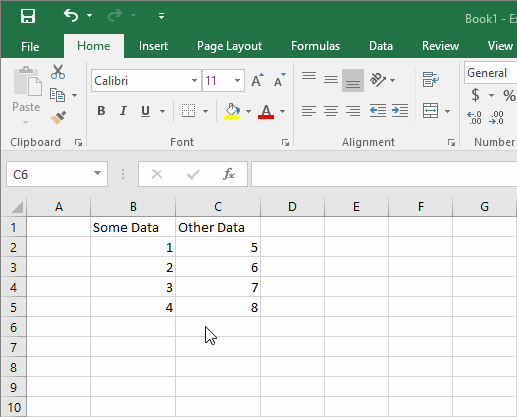The cell reference in this case is `B2:C5`.

OK, now that we have that basic understanding, let’s look at what relative references and absolute references are.## Relative Cell References

Relative cell references basically means that if you were to copy and paste a formula that had relative references in it, then the formula’s references would change, relative to where you copied from.

For example, if we had a formula in cell `B6` like:

``=SUM(B2:B5)``

And then we copied that formula to cell `C6`, the formula would change to:

``=SUM(C2:C5)``

Here’s an animated example: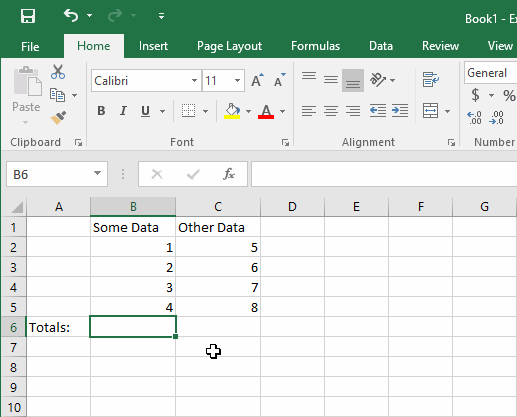The format of relative cell references is:

``[Cell Column Reference][Cell Row Reference] (i.e. B2)``

However, absolute cell references have some extra formatting. Let’s look into that and see what’s going on.

## Absolute Cell References

Let’s start by showing the format of absolute cell references. Going back to our previous example, if we set the formula in cell `B6` to be:

``=SUM(\$B2:\$B5)``

The difference is with the dollar sign `\$`. This is a special character for Excel formulas which will “lock” the cell reference that you are referring to. Here is the format:

``\$[Cell Column Reference]\$[Cell Row Reference] (i.e. \$B\$2)``

Notice that I put a dollar sign `\$` in front of both the column reference as well as the row reference. This is because you can lock either the column reference, the row reference, or both.

### What do you mean by “lock the reference”?

It’s easiest to show you with an example. Let’s go back to our example and say that `B6` contains this formula:

``=SUM(\$B2:\$B5)``

And then we’ll copy and paste cell `B6` to `C6` and let’s see what happens to the formula in `C6`.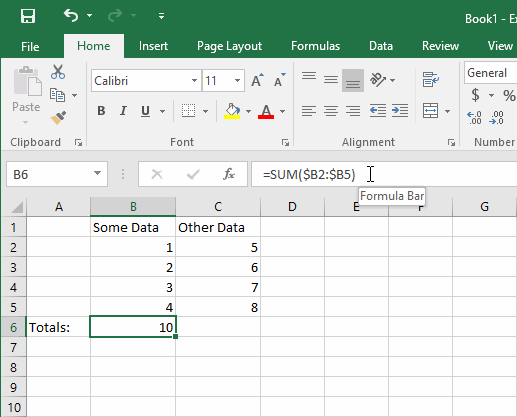The value in `C6` is the same as in `B6`, so what’s going on here?

Because the formula had `\$` in front of the column reference, then what happens is that the column’s reference is absolute, meaning that it will not change relative to where you pasted to, as shown above.

However, we didn’t lock the row reference, so what would happen if we copied `C6` to `C7`?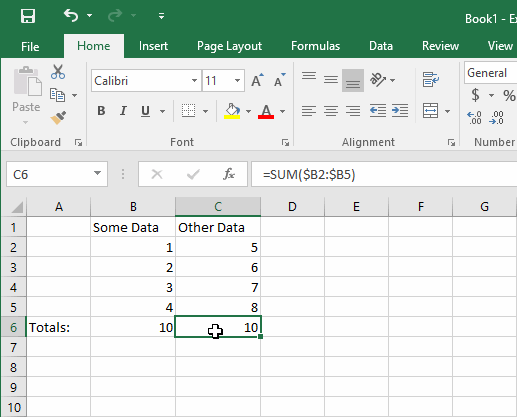The rows have adjusted in the formula in `C7`. Notice that they have adjusted one row down, which is one row relative to where you copied the formula from. So, we copied the cell `C6` and pasted one row down to `C7`, where the row references have adjusted themselves to move one row down along with you (hence, making them the relative references).

Now, you can make whatever column or row reference absolute or relative. You can even mix and match them if you like (and sometimes, it is helpful to do so in some formulas). Let me illustrate this for you with one more example.

Let’s change the formula in cell B6 to be:

``=SUM(\$B2:B\$5)``

So, the top-left cell reference is locking the column, and the bottom-right cell reference is locking the row. What do you think will happen if we copy and paste this formula one column to the right and one row down to cell `C7`?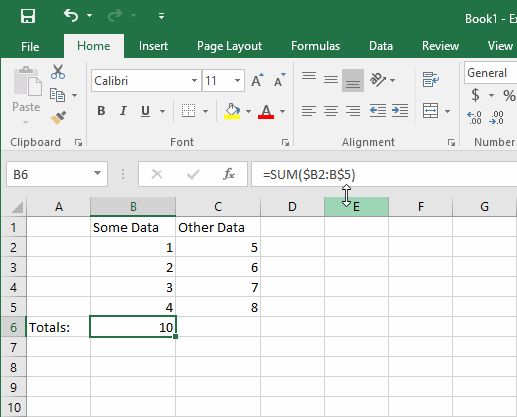The formula in `C7` changed into:

``=SUM(\$B3:C\$5)``

What happened?

When we copy and pasted one column to the right and one row down, the top-left cell reference of `\$B2` locked the column, but not the row. So the column stayed absolutely as column `B`, but the row changed relatively to row `3`. Similarly, the bottom-right cell reference that was `B\$5` changed to `C\$5` because the column was a relative reference and so moved relatively one column to the right, while the row absolutely stayed at `5`.

I know it’s a bit confusing at first, but try making some examples of your own and play around with it for a bit to get used to it.

## Keyboard shortcut to toggle between absolute and relative references

A nice trick that you can use is the F4 key on your keyboard to cycle through different reference modes in your formula.

The reference mode cycle goes like this (each time you press F4):

1. Rows and columns are relative
2. Rows and columns are absolute
3. Rows are absolute, columns are relative
4. Rows are relative, columns are absolute

If we go back to our simple formula of:

``=SUM(B2:B5)``

And if we edit the formula in the formula bar, we can highlight the top-left cell reference, the bottom-right cell reference, or both cell references and toggle through the different reference modes (absolute or relative) with the `F4` key. Here’s an illustration to make it a little more clear (NOTE: each time you see the cell reference changing, I’m pressing `F4` on my keyboard):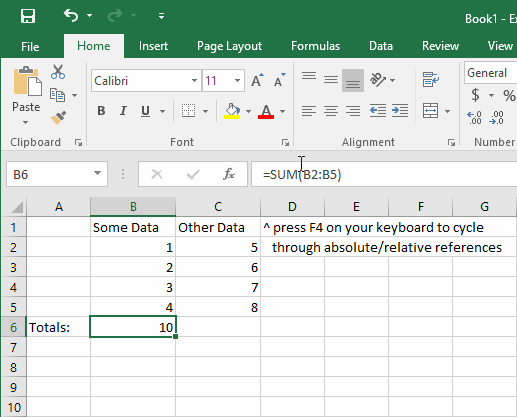I hope you have found this information useful. Please comment below if you have any questions or tips you’d like to share!

Wow, you read the whole article! You know, people who make it this far are true learners. And clearly, you value learning. Would you like to learn more about Excel? Please consider supporting me by buying me a coffee (it takes a lot of coffee to write these articles!).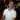Written by Joseph who loves teaching about Excel.